A Source Book in Chemistry, 1900-1950

Contents:
Author: Sören Peter Lauritz Sörensen  | Date: 1909

Show Summary
Sören Peter Lauritz Sörensen Chemistry

### HYDROGEN ION CONCENTRATION; PH.

The following selection is from pages 131–134 and 159–160 of Bio-chemische Zeitschrift 21, 131–200 (1909); the paper also appeared in Comptes rendus des Travaux du Laboratoire de Carlsberg 8, 1 (1909).

# Enzyme Studies II. The Measurement and Meaning of Hydrogen Ion Concentration in Enzymatic Processes

S. P. L. SÖRENSEN

### 1. Degree of Acidity—Hydrogen Ion Concentration

It is a well-known fact that the rate at which enzymatic splitting takes place depends among other things on the degree of acidity or alkalinity of the solution used. Usually—in fact, we may say always—the degree of acidity or alkalinity in enzymatic processes is calculated from the total amount of acid or base added and is expressed by this; it is not at all customary to call attention to the dissociation constant of the acid or base used; still more rarely is the ability of the solutions to bind acids or bases considered.

There can be no doubt that in such a case the ideas on the nature of solutions involved in the Arrhenius theory of electrolytic dissociation can be applied. If, for example, pepsin digestion takes place in a hydrochloric acid solution which is 0.1 normal with respect to the total content of hydrochloric acid, we cannot fail to consider that 0.1 normal hydrochloric acid is not fully dissociated and that the "actual degree of acidity" must rationally be considered the hydrogen ion concentration, because this is somewhat less than 0.1 normal. We must equally consider how much the solution of salts such as phosphates can exchange with the hydrochloric acid or what is the content of other substances which can influence the hydrogen ion concentration; specifically, we cannot foget that the substrate, in this special case an available protein, can bind acids. Since the hydrogen ion concentration of the solution is dependent only on the amount of free dissociated acid present, and, of course, since the amount of acid bound to the protein depends on the nature and amount of this substance, it is immediately clear that two solutions of which, for example, one contains 1 g. of protein, the other 5 g. in 100 cc of 0.1 N hydrochloric acid, and which, consequently, are of the same degree of acidity according to the usual method of expression, have very different "actual degrees of acidity," very different hydrogen ion concentrations.

These ideas of the effect of degree of acidity and hydrogen ion concentration on pepsin digestion are valid for all enzymatic processes. I have chosen pepsin-splitting as an example because this process takes place best at a greater hydrogen ion concentration and therefore the relationship is clearer and is also known to some extent. However, exactly the same considerations must also apply to enzymatic behavior which is found to be best in weakly acid, neutral, or alkaline solutions. In the last section of this paper we will give examples of the significance of hydrogen ion concentration in other enzymatic reactions, just as in pepsin digestion; the difference is found only in the order of magnitude of the hydrogen ion concentration with which we must reckon in different cases.

### 2. The Significance of Hydrogen Ion Concentration. The Hydrogen Ion Exponent

If we denote the concentration of hydrogen ions, of hydroxyl ions, and of water in a water solution by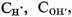and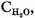then, as is well known, the following equation holds, by virtue of the law of chemical mass action.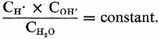Since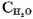for a somewhat dilute solution can be considered to be constant, then too the product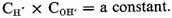This product, which usually, and also in this paper, is called the dissociation constant of water, at 18° equals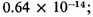in a series of measurements which have been carried out here in our laboratory and which are described in a later section, we have found the average value of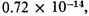or, written in another way, 10−14.14. As is easily seen, this value permits calculation of the hydrogen ion concentration of aqueous solutions if the concentration of the hydroxyl ion is known, or vice versa. Naturally, since the value for the dissociation constant of water is affected by errors and also the concentration of hydrogen ions is usually easier to determine more exactly than that of the hydroxyl ions, it is more rational, as H. Friedenthal has reported, so far as possible always to determine the hydrogen ion concentration of a solution and to introduce it into the calculation even when the solution has an alkaline reaction. This procedure will therefore be used in what follows since, for example, a solution whose normality based on hydrogen ions equals 0.01 is denoted by 0.01 N; it can be denoted briefly by 10−2 without using normalities. In the same way the hydrogen ion concentration of a solution which is based on hydroxyl ions, for example 0.01 normal, is given by 10−12.14, since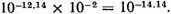Completely pure water and a truly neutral solution, using the same expression, will have a hydrogen ion concentration of 10−7.07, since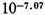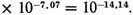The value of the hydrogen ion concentration will accordingly be expressed by the hydrogen ion based on the normality factor of the solution used, and this factor will have the form of a negative power of 10. Since in the following section I usually refer to this, I will explain here that I use the name "hydrogen ion exponent" and the designation PH for the numerical value of the exponents of this power. In the three examples given above, the PH will therefore be 2, 12.40, and 7.07, respectively. . . .

CP, which is based on the normality factor of the solution on hydrogen ions, or, in other words, means the number of gram atoms of hydrogen ion per liter, is less than 1 in all the cases treated in this paper, and can thus be placed equal to 10−P. For the number P, I have chosen the name "hydrogen ion exponent" and the written expression PH. By the hydrogen ion exponent (PH) of a solution we understand the Briggs logarithm of the reciprocal value of the normality factor of the solution based on the hydrogen ions.1

1 I have chosen the above definition of the hydrogen ion exponent because only rarely will it be necessary to take account of a hydrogen ion solution stronger than 1 normal. It will therefore usually be a positive number, and only in rare, exceptional cases will it be negative in dealing with a stronger hydrogen ion concentration than 1 normal.

Contents:

### Related Resources

None available for this document.

Title: A Source Book in Chemistry, 1900-1950

Select an option:

## Email Options

Title: A Source Book in Chemistry, 1900-1950

Select an option: